Looking for a way to refine your math lessons? It is tough to go this alone, so why not work together with your peers. Lesson Studies are a fantastic approach in working together as a team to share, develop, and refine great ideas in teaching math.

A great place to begin is by focusing on selecting an excellent math goal and task. This link can get you started and provide direction in implementing a lesson study with a team or other group of like-minded math teachers at your school.In 3rd grade, students are expected to generate simple equivalent fractions.

Example: 1/2 = 4/8 and 4/6 = 2/3.

Models should be used to help students identify equivalent fractions. Sentence strips or strips of paper cut into congruent rectangles can be used to model the idea of equivalency.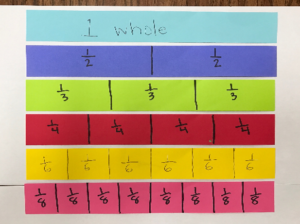Students will build conceptual understanding of equivalent fractions through the use of area and linear models. Number lines should be often used to model as it is an efficient strategy for finding equivalent fractions.As the standard states, students should be able to “explain why fractions are equivalent e.g, by using a visual fractional model”. The use of procedures or algorithms is not a 3rd grade expectation. Students will have more success and understanding when they can prove if two fractions are equal or not.

Learn Zillion – Recognize Equivalent Fractions Using Number Lines: https://learnzillion.com/lesson_plans/4739-recognize-equivalent-fractions-using-number-lines

Comparing using a number line –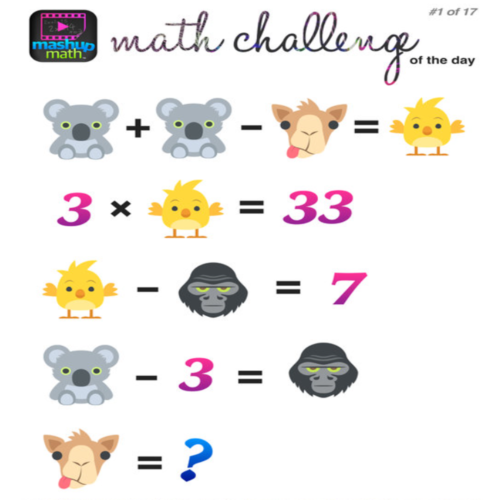Check out the website MashUp Math for awesome math challenges! These are great way to get students excited about math and stretch their algebraic thinking!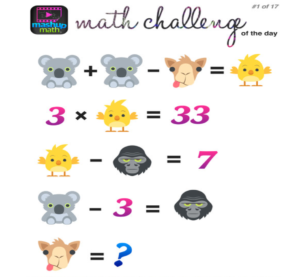In 3rd grade, students are expected to compare two fractions that have either the same numerator or the same denominator. When comparing fractions, it is important for students to understand the value of the fraction and consider both the numerator and denominator and their relationship to each other. So be cautious of teaching statements such as:

“The smaller the denominator, the larger the fraction.”

“The larger the denominator, the smaller the fraction.”

It is important for students to begin comparing fractions with a concrete representation, such as the area model to help them understand the size of the parts and number of parts.Using representations to compare fractions help students develop their number sense about fraction size. This awareness helps them to understand the strategies they use to compare whole numbers do not necessarily compare to fractions (1/2 is greater than 1/6 even though the whole number 6 is greater than 2). Through hands on experience, students will understand that the larger the digit in the denominator the smaller the piece.Make sure these symbols are used when comparing fractions:

< less than > greater than

= equal toThese Learn Zillion videos support students understanding of comparing fractions with the same denominator or numerator:

Compare fractions to the same whole: https://learnzillion.com/lesson_plans/7801-compare-fractions-to-the-same-whole

Compare fractions with the same numerator: https://learnzillion.com/lesson_plans/8217-compare-fractions-with-the-same-numerator-using-the-greater-than-and-less-than-symbolsCongratulations to the 5th grade winner of the Problem of the Month competition for December! The winner is Noah from Ms. Cannella’s classroom at Mitchell Elementary.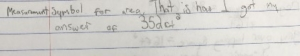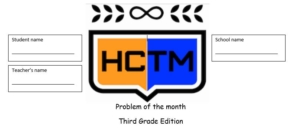Congratulations to the 3rd grade winner of the Problem of the Month competition for December! The winner is Nicholas from Ms. Steckman’s classroom at McKitrick Elementary.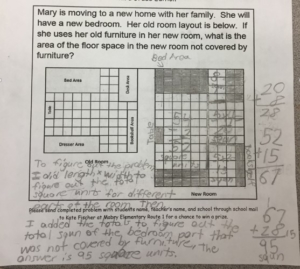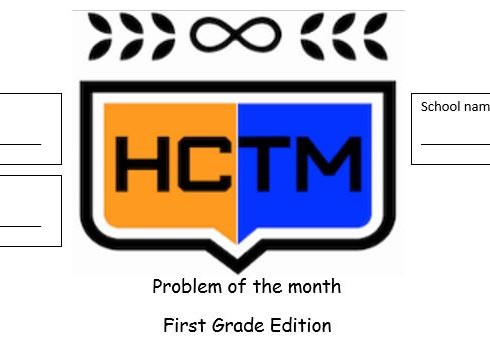Congratulations to the 1st grade winner of the Problem of the Month competition for December! The winner is Sagnik from Ms. Gordon’s classroom at Deer Park Elementary.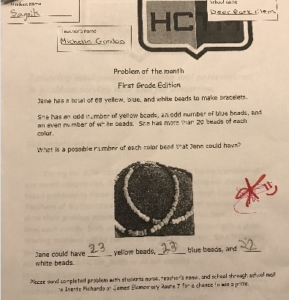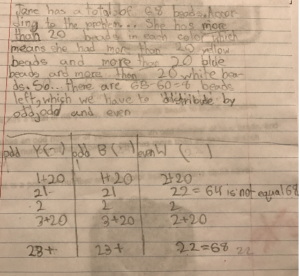Like most people, for my New Year resolution, I resolved to save more money!!! To accomplish this, I plan to save all my dimes in a Mason jar “bank.” My goal is to fill the mason jar completely!

Your task is help me determine how much money I will have saved if I fill the mason jar completely?

In the images below, you will see exactly one dollar in dimes in the jar.

According to the Standards for Mathematical Practices, students who, Make Sense of problems and persevere in solving them, “start by explaining to themselves the meaning of a problem and looking for entry points to its solution. They analyze givens, constraints, relationships, and goals. They make conjectures about the form and meaning of the solution and plan a solution pathway rather than simply jumping into a solution attempt.”

If I know that there is a dollar in the images above, what is the value of the dimes in the image below?

Questions to pose to students as they attempt to solve the problem:
What information do you need to solve this problem?
How are you going to use that information to help you solve?
How might knowing the first picture represents one dollar in dimes help you in solving the problem?
Is there more or less than 5 dollars in the jar right now? How do you know?

According to the SMP’s, “Mathematically proficient students check their answers to problems using a different method, and they continually ask themselves, ‘Does this make sense?'”

Now that you have some ideas about how to determine the value in the jar, I need to know, how much money will be in the jar if the jar is filled completely?

Happy Problem Solving!!!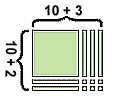MAFS.4.NBT.2.5 states that 4th graders should be able to:
Multiply a whole number of up to four digits by a one-digit whole number, and multiply two two-digit numbers, using strategies based on place value and the properties of operations Illustrate and explain the calculation by using equations, rectangular arrays, and/or area models.

When introducing multi-digit multiplication, the tendency is to dive right in to the standard algorithm. While the standard algorithm is an efficient strategy, it is very procedural and many kids copy performing the steps without understanding the process, which can result in misconceptions that are difficult to undo. A great approach for building conceptual understanding is to begin with base-ten models, and then connect those models to the partial products method and area model. This ensures that students have an understanding of all parts of the standard.

You can see from the following examples of 324 x 6 the similarities in the three methods. The area model is just a pictorial representation of the base-ten model, while partial products is a more abstract way of recording both strategies. All strategies are rooted in the student’s understanding of place value. The standard algorithm is actually a short-cut method of partial products, and fluency with the standard algorithm is a 5th grade standard. (https://www-k6.thinkcentral.com/content/hsp/math/hspmath/na/gr3-5/itools_intermediate_9780547274058_/basetenblocks.html virtual base-ten blocks were used to create the base-ten model.)Students see that to multiply 324 x 6, they actually multiply 6 times the ones, 6 times the tens, and 6 times the hundreds. These strategies allow students to break larger problems down so they can work with their basic facts and multiples of 10 and 100.

The area model is a great opportunity to make connections to arrays and students’ experience in 3rd grade with area.

All three strategies can also be applied when multiplying two 2-digit numbers, as shown in the representations of 12×13 shown below.
🙂If your students are struggling with using Think Addition to subtract, check out this video!Are your students still struggling with counting objects? Check out this quick video!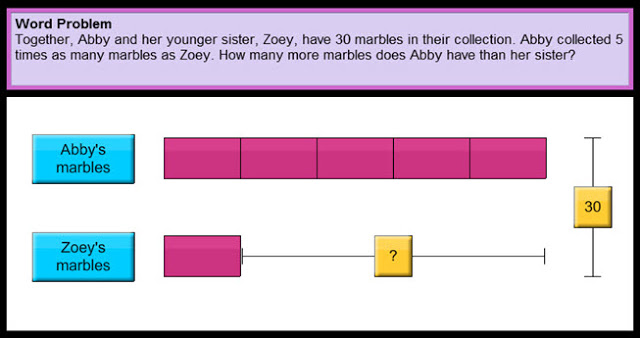Using simple bar models, often referred to as the “Singapore method”, to represent the mathematical relationships in comparison word problems helps students organize their thinking to more easily make sense of the problems and generate equations. Drawing bar models provides students with a strategy for visualizing abstract word problems in a more concrete way.

The videos below demonstrates how the bar model can be used to help make sense of multiplicative comparison problems. The tutorials use the “Thinking Blocks” virtual tool which can be found at http://www.mathplayground.com/thinkingblocks.html. This website has a variety of tutorials on modeling different types of comparison word problems, including those with two-steps. This tool not only has the benefits of supporting students with visualizing word problems, but it also provides them opportunities to manipulate models on the computer.

“>

“>Check out the video below for a quick and easy way to reinforce mathematics concepts on a number line.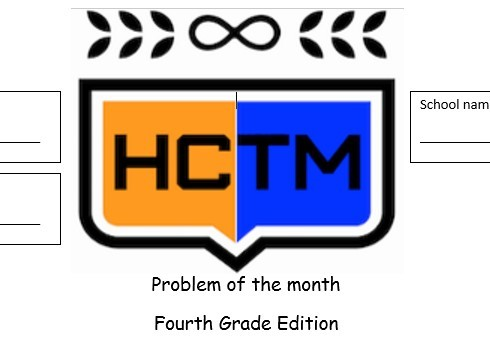What math do you see in this picture?According to the Standards for Mathematical Practices, “Mathematically proficient students make sense of quantities and their relationships in problem situations.”

How might students make sense of the quantities in this picture?
What math might they identify?

Happy Problem Solving!!!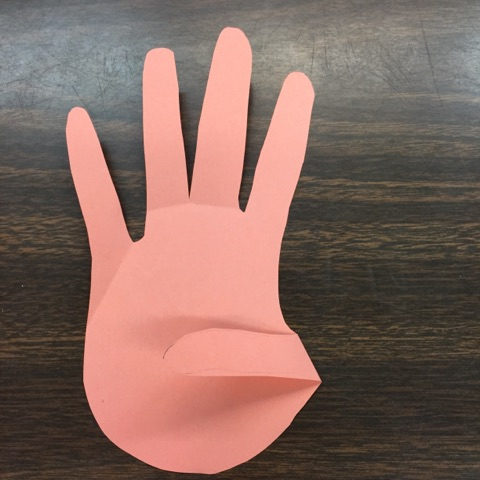Ahhh you had two weeks off for Winter Break and you are returning refreshed and ready to kick off the 2017 school year! Pretty soon 18 little minds will reenter your classroom! 17 days off of school means that it is an excellent time to reestablish classroom norms and expectations.

One way to review expectations while reinforcing number combinations is through the “Give me” strategy. Many teachers are familiar with the “Give me 5” strategy where students freeze and place their attention on the teacher or speaker.

A twist on this activity is for the teacher to say “Give me 5” but only hold up 3 fingers like in the image below.Students think about what other number combined with 3 makes 5. Since 2 is the addend that when combined with 3 makes a sum of 5, students should put 2 fingers in the air like in the image below.
***Note at this point in the year students have explored different combinations for making numbers, they have not formally been introduced to the idea that these number combinations are addends***To reinforce the number combinations ask students questions such as:
Why did you put up ___ fingers?
How did you know to put up ___ fingers?

This strategy could be extended to any number combination.
The teacher could say, “Give me 10” and hold up 1 finger in the air.Students would respond by holding up 9 fingers because the addends 1 and 9 make ten.
***Teacher Tip for managing materials: If you want students hands to be free of materials, select an addend that requires them to use both hands (any addend 6 or greater).Another twist on the strategy is for the teacher to hold up a sum and the students create the addends with their hands in the air. For example, a teacher might say, “What two numbers combined make 3 ?”If the sum is 3, students would hold up the addends 2 and 1Be creative!!! Think about different ways you can reinforce behavior and math at the same time!!!